Custom Test allows you to test a custom hypothesis. Refer to Custom Test in Standard Least Squares Report and Options for details on custom tests.
Contrast allows you to test for differences in levels within a variable. If a contrast involves a covariate, you can specify the value of the covariate at which to test the contrast.
Initial Contrast Window
Choose spine, the variable of interest, and click Go.
Contrast Specification Window
Click Done.
Contrast Report
Inverse Prediction is used to predict an X value, given specific values for Y and the other X variables. This can be used to predict continuous variables only. For more details about Inverse Prediction, see Inverse Prediction in Standard Least Squares Report and Options.
Covariance of Estimates produces a covariance matrix for all the effects in a model. The estimated covariance matrix of the parameter estimator is given by
Σ = -H-1
where H is the Hessian (or second derivative) matrix evaluated using the parameter estimates on the last iteration. Note that the dispersion parameter, whether estimated or specified, is incorporated into H. Rows and columns corresponding to aliased parameters are not included in Σ.
Correlation of Estimates produces a correlation matrix for all the effects in a model. The correlation matrix is the normalized covariance matrix. That is, if σij is an element of Σ, then the corresponding element of the correlation matrix is σij/σiσj, where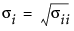Profiler brings up the Profiler for examining prediction traces for each X variable. Details on the profiler are found in Profiler in Standard Least Squares Report and Options.
Contour Profiler brings up an interactive contour profiler. Details are found in the Profilers book.
Surface Profiler brings up a 3-D surface profiler. Details of Surface Plots are found in the Profilers book.
Diagnostic Plots is a submenu containing commands that allow you to plot combinations of residuals, predicted values, and actual values to search for outliers and determine the adequacy of your model. Deviance is discussed above in Model Selection and Deviance. The following plots are available:
 • Studentized Deviance Residuals by Predicted
 • Studentized Pearson Residuals by Predicted
 • Deviance Residuals by Predicted
 • Pearson Residuals By Predicted
 • Actual by Predicted
 • Regression Plot
Note: Regression Plot is available only when there is one continuous predictor and no more than one categorical predictor.
 • Linear Predictor Plot
Note: Linear Predictor Plot is a plot of responses transformed by the inverse link function. This plot is available only when there is one continuous predictor and no more than one categorical predictor.
Model Dialog shows the completed launch window for the current analysis.
Effect Summary shows the interactive Effect Summary report that allows you to add or remove effects from the model. See Effect Summary Report.
Save Columns is a submenu that lets you save certain quantities as new columns in the data table. Formulas for residuals are shown in Residual Formulas.
 Prediction Formula Saves the formula that predicts the current model. Predicted Values Saves the values predicted by the current model. Mean Confidence Interval Saves the 95% confidence limits for the prediction equation. The confidence limits reflect variation in the parameter estimates. Save Indiv Confid Limits Saves the confidence limits for a given individual value. The confidence limits reflect variation in the error and variation in the parameter estimates. Deviance Residuals Saves the deviance residuals. Pearson Residuals Saves the Pearson residuals. Studentized Deviance Residuals Saves the studentized deviance residuals. Studentized Pearson Residuals Saves the studentized Pearson residuals. (JSL only) Parametric Formula Saves the parametric formula using JSL: fit model object <
 Residual Type Formula Deviance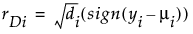Studentized Deviance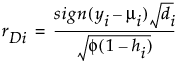Pearson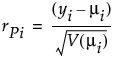Studentized Pearson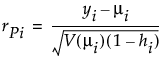where (yiμi) is the raw residual, sign(yiμi) is 1 if (yiμi) is positive and -1 if (yiμi) is negative, di is the contribution to the total deviance from observation i, φ is the dispersion parameter, V(μi) is the variance function, and hi is the ith diagonal element of the matrix We(1/2)X(X'WeX)-1X'We(1/2), where We is the weight matrix used in computing the expected information matrix. For additional information regarding residuals and generalized linear models, see “The GENMOD Procedure” in the SAS/STAT User Guide documentation.